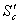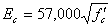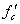# 1993 AASHTO Rigid Pavement Structural Design

Empirical equations are used to relate observed or measurable phenomena with outcomes.  There are many different types of empirical equations available today but this section will present the 1993 AASHTO Guide basic design equation for rigid pavements as an example.  This equation is widely used and has the following form (see Figure 6.5 for the nomograph form)(these variables will be further explained in Inputs)

 where: W18 equals predicted number of 80 kN (18,000 lb.) ESALs ZR equals standard normal deviate So equals combined standard error of the traffic prediction and performance prediction D equals slab depth (inches) pt equals terminal serviceability index ΔPSI equals difference between the initial design serviceability index, po, and the design terminal serviceability index, ptequals modulus of rupture of PCC (flexural strength) Cd equals drainage coefficient J equals load transfer coefficient (value depends upon the load transfer efficiency) Ec equals Elastic modulus of PCC k equals modulus of subgrade reaction

This equation is not the only empirical equation available but it does give a good sense of what an empirical equation looks like, what factors it considers and how empirical observations are incorporated into an equation.  The rest of this section will discuss the specific assumptions, inputs and outputs associated with the 1993 AASHTO Guide flexible pavement empirical design equation.  The following subsections discuss:

• assumptions
• inputs
• outputs

### Assumptions

From the AASHO Road Test, equations were developed which related loss in serviceability, traffic, and pavement thickness.  These equations were developed for the specific conditions of the AASHO Road Test and therefore involved some significant limitations:

• The equations were developed based on the specific pavement materials and roadbed soil present at the AASHO Road Test.
• The equations were developed based on the environment at the AASHO Road Test only.
• The equations are based on an accelerated two-year testing period rather than a longer, more typical 20+ year pavement life. Therefore, environmental factors were difficult if not impossible to extrapolate out to a longer period.
• The loads used to develop the equations were operating vehicles with identical axle loads and configurations, as opposed to mixed traffic.
• For JPCP and JRCP, all transverse joints were the same spacing. JPCP was 4.6 m (15 ft) and JRCP was 12.2 m (40 ft). All
transverse joints used dowel bars.
• All PCC was of the same mix design and used the same aggregate and portland cement.

In order to apply the equations developed as a result of the AASHO Road Test, some basic assumptions are needed:

• The characterization of subgrade support may be extended to other subgrade soils by an abstract soil support scale.
• Loading can be applied to mixed traffic by use of ESALs.
• Material characterizations may be applied to other surfaces, bases, and subbases by assigning appropriate values.
• The accelerated testing done at the AASHO Road Test (2-year period) can be extended to a longer design period.

When using the 1993 AASHTO Guide empirical equation or any other empirical equation, it is extremely important to know the equation’s limitations and basic assumptions.  Otherwise, it is quite easy to use an equation with conditions and materials for which it was never intended.  This can lead to invalid results at the least and incorrect results at the worst.

### Inputs

The 1993 AASHTO Guide equation requires a number of inputs related to loads, pavement structure and subgrade support.  These inputs are:

• Reliability.  The reliability of the pavement design-performance process is the probability that a pavement section designed using the process will perform satisfactorily over the traffic and environmental conditions for the design period (AASHTO, 1993).  In other words, there must be some assurance that a pavement will perform as intended given variability in such things as construction, environment and materials.  The ZR and So variables account for reliability.
• PCC elastic modulus.  If no value is known, the PCC elastic modulus (Ec) can be estimated from relationships such as the following:where: Ec equals PCC elastic modulusequals PCC compressive strength

If no compressive strength data are available (or cannot be assumed), assume Ec = 27,500 MPa (4,000,000 psi), which corresponds to a compressive strength of 34.5 MPa (5000 psi).

• PCC modulus of rupture (flexural strength). The modulus of rupture (S’c) is typically obtained from a flexural strength test.
• Slab depth.  The pavement structure is best characterized by slab depth (D). The number of ESALs a rigid pavement can carry over its lifetime is very sensitive to slab depth. As a general rule, beyond about 200 mm (8 inches) the load carrying capacity of a rigid pavement doubles for each additional 25 mm (1 inch) of slab thickness.
• Drainage coefficient. Rigid pavement is assigned a drainage coefficient (Cd) that represents the relative loss of strength due to its drainage characteristics and the total time it is exposed to near-saturation moisture conditions.  Generally, quick-draining layers that almost never become saturated can have coefficients as high as 1.2 while slow-draining layers that are often saturated can have drainage coefficients as low as 0.80.  If subsurface drainage is expected to be a problem, positive drainage measures should be taken.  In general, the use of drainage coefficients to overcome poor drainage conditions is not recommended (i.e. more slab thickness does not necessarily solve water-related problems).  Because of the peril associated with its use, often times the drainage coefficient is neglected (i.e., set as Cd = 1.0).
• Serviceable life.  The difference in present serviceability index (PSI)between construction and end-of-life is the serviceability life.  The equation compares this to default values of 4.2 for the immediately-after-construction value and 1.5 for end-of-life (terminal serviceability).  Typical values used now are:
• Post-construction: 4.0 – 5.0 depending upon construction quality, smoothness, etc.
• End-of-life (called “terminal serviceability” and designated “pt“): 1.5 – 3.0 depending upon road use (e.g., interstate highway, urban arterial, residential)
• Load transfer coefficient (J Factor).  This accounts for load transfer efficiency.  Essentially, the lower the J Factor the better the load transfer.  The J Factor for the AASHO Road Test was estimated to be 3.2.  Typical J factor values are as shown below.
 Condition J Factor Undoweled PCC on crushed aggregate surfacing 3.8 Doweled PCC on crushed aggregate surfacing 3.2 Doweled PCC on HMA (without widened outside lane) and tied PCC shoulders 2.7 CRCP with HMA shoulders 2.9 – 3.2 CRCP with tied PCC shoulders 2.3 – 2.9
• Modulus of subgrade reaction.  The modulus of subgrade reaction (k) is used to estimate the “support” of the PCC slab by the layers below.  Usually, an “effective” k (keff) is calculated which reflects base, subbase and subgrade contributions as well as the loss of support that occurs over time due to erosion and stripping of the base, subbase and subgrade.  Typically, large changes in keff have only a modest impact on PCC slab thickness.

### Outputs

The 1993 AASHTO Guide equation can be solved for any one of the variables as long as all the others are supplied.  Typically, the output is either total ESALs or the required slab depth (D).  In design, the rigid pavement equation described in this chapter is typically solved simultaneously with the rigid pavement ESAL equation.  The solution is an iterative process that solves for ESALs in both equations by varying the slab depth (D).  The solution is iterative because the slab depth (D) has two key influences:

1. The slab depth (D) determines the total number of ESALs that a particular pavement can support.  This is evident in the rigid pavement design equation presented in this section.
2. The slab depth also determines what the equivalent 80 kN (18,000 lb.) single axle load is for a given load.

Therefore, the slab depth (D) is required to determine the number of ESALs to design for before the pavement is ever designed.  The iterative design process usually proceeds as follows:

1. Determine and gather rigid pavement design inputs (ZR, So, DPSI, pt, Ec, S’c, J, Cd and keff).
2. Determine and gather rigid pavement ESAL equation inputs (Lx, L2x, G)
3. Assume a slab depth (D).
4. Determine the equivalency factor for each load type by solving the ESAL equation using the assumed slab depth (D) for each load type.
5. Estimate the traffic count for each load type for the entire design life of the pavement and multiply it by the calculated ESAL to obtain the total number of ESALs expected over the design life of the pavement.
6. Insert the assumed slab depth (D) into the design equation and calculate the total number of ESALs that the pavement will support over its design life.
7. Compare the ESAL values in #5 and #6.  If they are reasonably close (say within 5 percent) use the assumed slab depth (D).  If they are not reasonably close, assume a different slab depth (D), go to step #4 and repeat the process.

## Design Utility

This design utility solves the 1993 AASHTO Guide basic design equation for rigid pavements. It also supplies some basic information on variable descriptions, typical values and equation precautions.

1993 AASHTO Rigid Pavement Structural Design

Footnotes    (↵ returns to text)
1. AASHTO Guide for Design of Pavement Structures.  American Association of State Highway and Transportation Officials.  Washington, D.C.